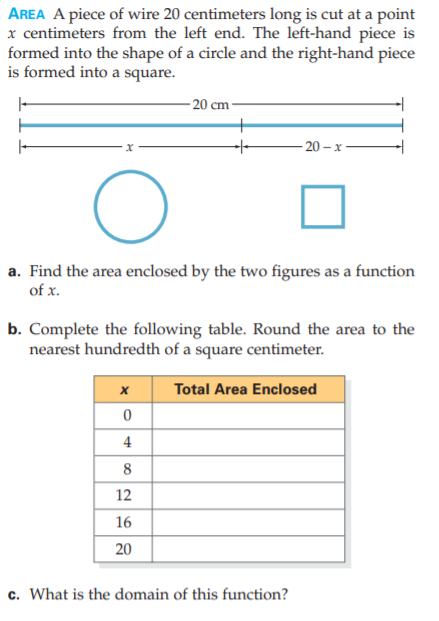### Still have math questions?

Algebra
QuestionAREA A piece of wire $$20$$ centimeters long is cut at a point

$$x$$ centimeters from the left end. The left-hand piece is formed into the shape of a circle and the right-hand piece is formed into a square. a. Find the area enclosed by the two figures as a function of $$x$$ . b. Complete the following table. Round the area to the nearest hundredth of a square centimeter. c. What is the domain of this function?

$$\left. \begin{array} { l } { \text { a. } A ( x ) = ( \frac { 1 } { 4 \pi } + \frac { 1 } { 16 } ) x ^ { 2 } - \frac { 5 } { 2 } x + 25 \quad \text { b. } 25,17.27,14.09,15.46,21.37,31.83 } \\ { \text { c. } [ 0,20 ] } \end{array} \right.$$